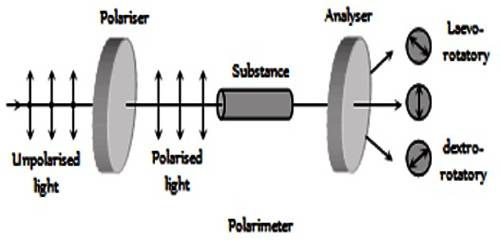Chemistry

# Optical ActivityOptical Activity is a substance is said to be optically active if it can rotate the plane polarization of polarized light. It is the property (displayed by solutions of some compounds, notably many sugars) of rotating the plane of polarization of plane-polarized light. Ordinary light is believed to consist of electromagnetic waves, the oscillation taking place in all planes perpendicular to the direction of propagation. If, however, the light is passed through a Nicol prism the oscillations take place in a single plane and the light is said to be plane polarized and the plane in which oscillations take place is called the plane of polarization. A schematic diagram of the plane polarized light and its rotation is shown in the figure. When solutions of substances like sucrose, glucose etc. are placed in a beam of plane polarized light, the plane of polarization is found to be rotated either to the right or to the left. If it is rotated to the right the substance is said to be dextro-rotatory, while if the plane is rotated to the left the substance is laevo-rotatory. The extent of rotation is measured in angles and is determined with a polarimeter. The property of rotation of the plane of polarization is also shown by pure solids and liquids.The extent of the optical rotation of a substance depends on a number of factors, viz., the wavelength of light, the temperature, the length of the column through which the light passes, the concentration, if the substance is in solution. The optical activity of a pure substance is usually expressed in terms of specific rotation, [α], given by the relation,

[α]sD = α/lρ

where the subscript D and superscript ‘t’ on a represent the wave-length (sodium-D line) of light used and temperature respectively, α is the angle of rotation when the light passes through a column  of length ‘l’ (expressed in decimeters) of the liquid density ρ, in g (mL)-1. For a solution, the relationship is written as

[α]lD = α/Lc … … (1)

where ‘c’ is the concentration in g (mL)-1 of the solution. If l = 1.0 dm and c = 1.0 g (mL)-1, equation (1) can be written as,

[α]lD = α

Thus the specific rotation may be defined as,

The angle through which the plane of Polarization of polarized light is rotated when one gram of substance is dissolved in 1.0 mL of solution and the length of the solution through which the light passes is 1.0 dm.